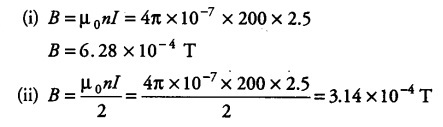# A solenoid of length 50 cm having 100 turns carries a current of 2.5 A

A solenoid of length 50 cm having 100 turns carries a current of 2.5 A. Find the magnetic field (i) in the interior of the solenoid, (ii) at one end of the solenoid.

Here, I = 2.5 A n = 100/0.50= 200 mwhy we divided 100 by 0.50 and why not 50 ??